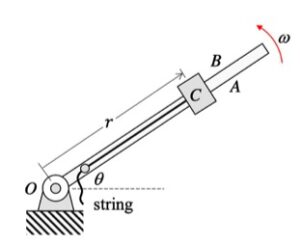# Homework H.4.A## 10 thoughts on “Homework H.4.A”

1.hu665 says:

since theta is unknown, do we leave theta in the equation?

1.CMK says:

Please note that the motion of C is in a HORIZONTAL plane. Therefore, theta is not a factor in either the forces acting on C or in the kinematics of the motion of C. You will find that the value of the angle is not a factor in this problem. Just work it out, and you will see.

1.lee3581 says:

Could you clarify what you mean by that the motion of C is in a Horizontal plane? I was using polar coordinates, so the weight force would need the theta to be described as sin and cos theta.

2.Chick-fil-A Official says:

Is the string pulling the block c to the left? so the block c is moving to the left on the arm?

1.CMK says:

Chick-fil-A Official: Since r_dot < 0, the value of r is decreasing in time. Therefore, block C moves toward O along the arm.

3.sophiek says:

Are we only evaluating 2 forces or should there be 3?

1.CMK says:

Sofiek: It looks like two forces: one force due to the cable, and one force due to the arm. About which third force are you asking?

1.Cullen Barber says:

Tina mentioned in class that you cannot neglect gravity, even though it is in the z direction, but Fg should just equal the normal force in the z, so it is neglected?

1.rgodjali says:

I believe it is

2.sophiek says:

I was wondering if gravity is negligible. Thank you all for clarifications.